## TRIANGLES: Finding Unknown Angles

Practice Unlimited Questions

#### 1. Angles of an isosceles triangle

Make an isosceles triangle as below.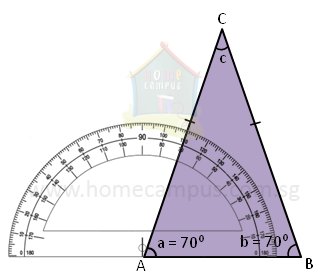Now, using a protractor, measure the 2 angles opposite its equal sides. What do you notice?

In the figure above,
∠a  =  70°
∠b  =  70°

The angles opposite the equal sides of the isosceles triangle are equal.

#### 2. Angles of an equilateral triangle

Make an equilateral triangle as below with equal sides.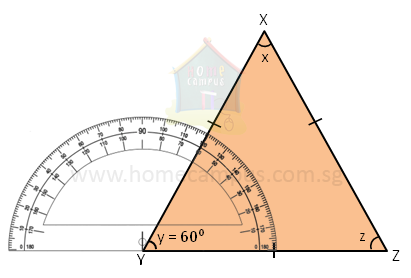Now, using a protractor, measure all the angles of the triangle. What do you notice?

In the figure above,
∠x  =  60°
∠y  =  60°
∠z  =  60°

All 3 angles of an equilateral triangle are equal. Each angle is 60°.

#### 3. Angles of a right-angled triangle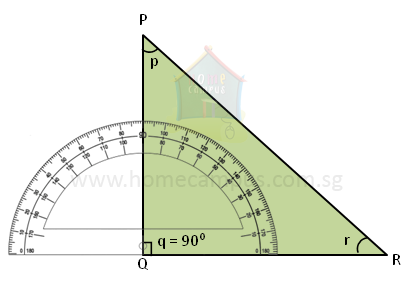Consider the right-angled triangle above.

∠p  +  ∠r  +  ∠q  =  180°
∠p  +  ∠r  +  90°  =  180°
∠p  +  ∠r  =  90°

The sum of the other two angles of a right-angled triangle is 90°.

#### 4. In the triangle below, AB = BC and ∠ABC = 80°. Find the other two angles.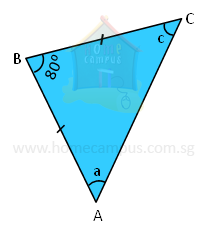180°  −  80°  =  100°
100°  ÷  2  =  50°

∠a  =  50°
∠c  =  50°

The sum of angles of a triangle is 180°.
Since AB = BC, ΔABC is an isosceles triangle.
So, ∠a = ∠c.

#### 5. In triangle PQR below, PQ = QR and ∠QPR = 45°. Is ΔPQR a right-angled triangle?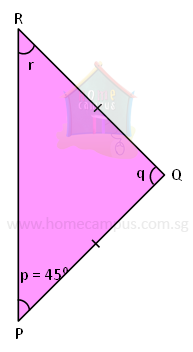∠p  =  ∠r
∠r  =  45°
∠q  =  180°  −  45°  −  45°  =  90°
Since, PQ = QR, ΔPQR is an isosceles triangle, and ∠p = ∠r.
Since, one of the angles of triangle PQR is 90°, ΔPQR is a right-angled triangle.

It is, in fact, a right-angled isosceles triangle (as one of its angles is 90° and two of its sides are equal).